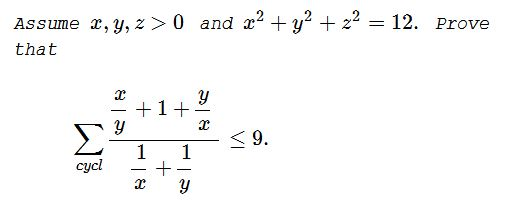# Dan Sitaru's Cyclic Inequality In Three Variables with Constraints II

### Problem### Solution 1

We first prove that

For $x,y\gt 0,$

$\displaystyle \frac{x^2+xy+y^2}{x+y}\le\frac{3}{2}\sqrt{\frac{x^2+y^2}{2}}.$

For a proof, we have a sequence of equivalent inequalities:

\displaystyle\begin{align} &\frac{(x^2+xy+y^2)^2}{(x+y)^2}\le\frac{9}{8}(x^2+y^2)\\ &8(x^2+xy+y^2)^2\le 9(x^2+y^2)(x+y)^2\\ &x^4+y^4-6x^2y^2+2x^3y+2xy^3\ge 0\\ &(x^2-y^2)^2+2x^3y-2x^2y^2+2xy^3-2x^2y^2\ge 0\\ &(x^2-y^2)^2+2x^2y(x-y)-2xy^2(x-y)\ge 0\\ &(x^2-y^2)^2+2xy(x-y)^2\ge 0, \end{align}

which is true since $x,y\gt 0.\,$ Using that and the Cauchy-Schwarz inequality,

\displaystyle \begin{align} \sum_{cycl}\frac\frac{x}{y}+1+\frac{y}{x}}\frac{1}{x}+\frac{1}{y}}&=\sum_{cycl}\frac{x^2+xy+y^2}{x+y}\\ &\le \frac{3}{2}\sum_{cycl}\sqrt{\frac{x^2+y^2}{2}}\\ &\le\frac{3}{2}\sqrt{(1^2+1^2+1^2)\sum_{cycl}\frac{x^2+y^2}{2}}\\ &=\frac{3}{2}\sqrt{3\sum_{cycl}x^2}\\ &=\frac{3}{2}\sqrt{3\cdot 12}=\frac{3}{2}\cdot 6=9. \end{align

### Solution 2

Starting with $(x-y)^2(x^2+4xy+y^2)\,$ we arrive through a chain of equivalent inequalities to $\displaystyle \frac{x^2+xy+y^2}{x+y}\le\frac{3}{2}\sqrt{\frac{x^2+y^2}{2}},\,$ proceeding from which we apply Jensen's inequality:

$\displaystyle \sum_{cycl}\sqrt{\frac{x^2+y^2}{2}}\le\sqrt{3\sum_{cycl}\frac{x^2+y^2}{2}}=6.$

### Acknowledgment

Dan Sitaru has kindly posted the above problem of his from the Romanian Mathematical Magazine at the CutTheKnotMath facebook page and later emailed me his solution (Solution 1) of the problem in a LaTeX file. Solution 2 is by Leo Giugiuc.